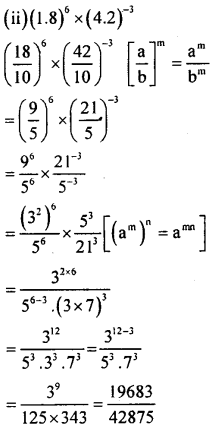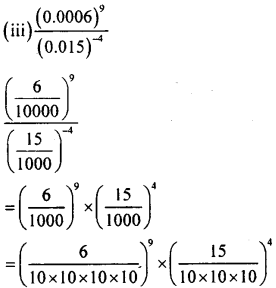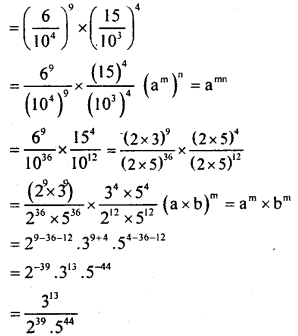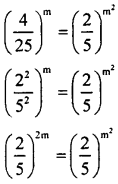# KSEEB Solutions for Class 8 Maths Chapter 10 Exponents Ex 10.6

Students can Download Maths Chapter 10 Exponents Ex 10.6 Questions and Answers, Notes Pdf, KSEEB Solutions for Class 8 Maths helps you to revise the complete Karnataka State Board Syllabus and score more marks in your examinations.

## Karnataka Board Class 8 Maths Chapter 10 Exponents Ex 10.6

Question 1.
Simplify
(i). $$\left(\frac{2}{3}\right)^{8} \times\left(\frac{6}{4}\right)^{3}$$
(ii). (1.8)6 × (4.2)-3
(iii). $$\frac{(0.0006)^{3}}{(0.015)^{-4}}$$Question 2.
Can it happen that for some integer m ≠ 0 $$\left(\frac{4}{25}\right)^{m}$$ = $$\left(\frac{2}{5}\right)^{\mathrm{m}^{2}}$$ ?∴ m2 = 2m
m.m=2m
m=2
It can happen if m=2

Question 3.
Find all positive integers m, n such that (3m)n = 3m × 3n